# Digital Electronics MCQ Level- 1

## 10 Questions MCQ Test Solid State Physics, Devices & Electronics | Digital Electronics MCQ Level- 1

Description
Attempt Digital Electronics MCQ Level- 1 | 10 questions in 30 minutes | Mock test for IIT JAM preparation | Free important questions MCQ to study Solid State Physics, Devices & Electronics for IIT JAM Exam | Download free PDF with solutions
QUESTION: 1

### Figure-I shows a logic circuit with two inputs A and B and output C. The voltage incorrect waveform of A, B and C are shown in figure-II. The logic circuit is :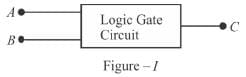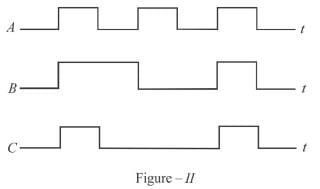Solution:

From the waveform A, B and C, it is clear that output is 1, if both B and A are 1 otherwise zero.
C = A . B
The correct answer is: AND gate

QUESTION: 2

### In Boolean Algebra,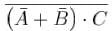will be equal to.

Solution: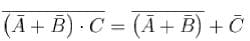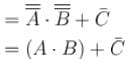The correct answer is: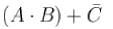QUESTION: 3

### When an input electrical signal A = 10100 is applied to a NOT gate, its output signal  is :

Solution:

NOT gate implies output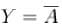where A is the input.

QUESTION: 4

The output Y from the following logic gate circuit will be :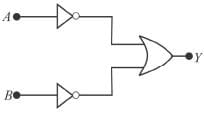Solution: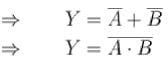The correct answer is: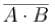QUESTION: 5

The Boolean expression of the output Y in terms of the inputs A and B for the circuit shown in the following figure is.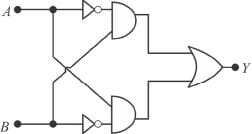Solution:

Equivalent circuit is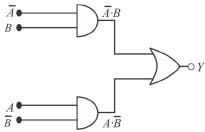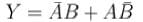The correct answer is: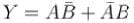QUESTION: 6

The binary number 110000111101 corresponds to a hexadecimal number.

Solution:

Hexadecimal is written with 16 numbers starting from 0, i.e., 0, 1,2, 3,...14, 15. The number 10 → A; 11 → B; 12 → C; 13 → D; 14 → E; 15 → F in place of two digits. To convert binary number in hexadecimal make the pairs of four numbers starting from right. Then convert them in decimal then hexadecimal e.g. (110000111101) ⇔ [(1100) (0011) (1101)2] in binary number ⇔ [(12) (3) (13) ] in decimal
= C3D is the equivalent hexadecimal number

QUESTION: 7

Figure I shows a two input type of logic circuit where A and B are the inputs and C is the output. The truth table of the logic gate is shown in Figure II.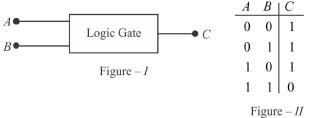Solution:

NAND implies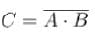QUESTION: 8

Which one of the following logic gate does the given truth table represent?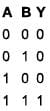Solution:

⇒ AND gate implies Y=A.B.

QUESTION: 9

The given figure shows the wave forms for two inputs A and B and that for the output V of logic circuit. The logic circuit is :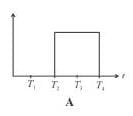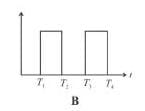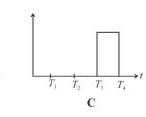Solution:

From the response of A, B and C, it is clear that C = A.B, hence, an AND gate.
The correct answer is: AND gate

QUESTION: 10

The truth table for the input NAND gate is given by :

Solution:

NAND gate is given as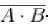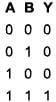Use Code STAYHOME200 and get INR 200 additional OFF Use Coupon Code